# Deep Mutual Learning

Posted by JoselynZhao on July 24, 2020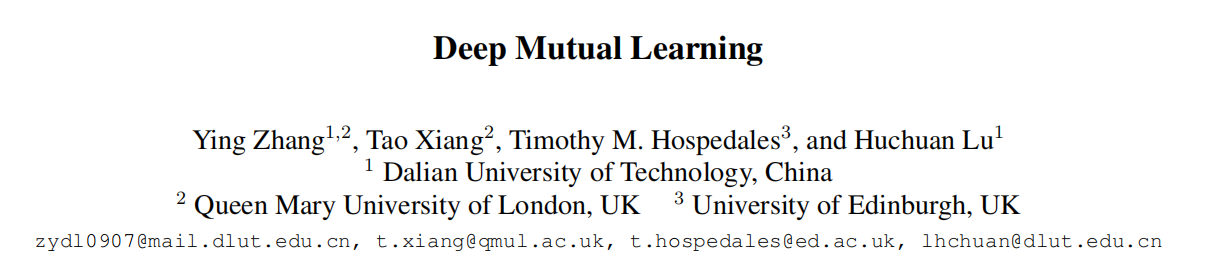文章提出了一种简单且普遍适用的方法，通过与同辈和相互蒸馏进行的队列训练来改善深层神经网络的性能。 通过这种方法，我们可以获得比那些强大但静态的teacher提炼的网络性能更好的紧凑网络。 DML的一种应用是获得紧凑，快速和有效的网络。 我们还表明，这种方法也有望改善大型强大网络的性能，并且可以将以此方式训练的网络队列作为一个整体进行组合，以进一步提高性能。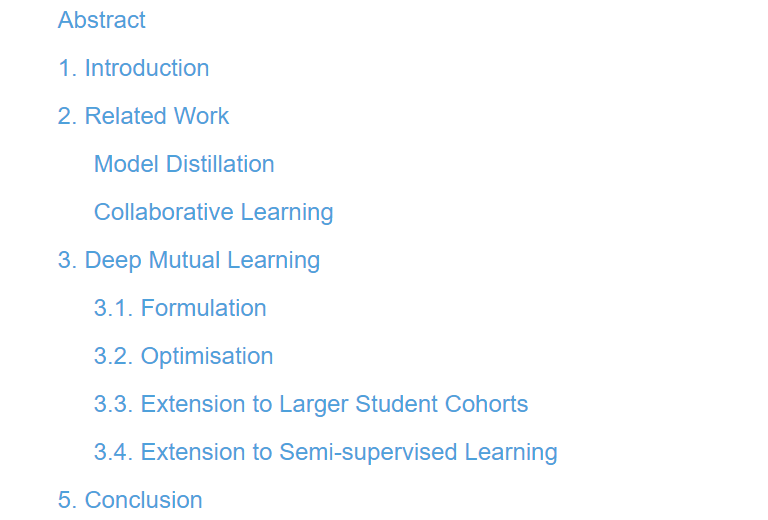# Abstract

Model distillation is an effective and widely used technique to transfer knowledge from a teacher to a student network.

Different from the one-way transfer between a static pre-defined teacher and a student in model distillation, with DML, an ensemble of students learn collaboratively and teach each other throughout the training process.

# 1. Introduction

Distillation-based model compression relates to the observation [3, 1] that small networks often have the same representation capacity as large networks.

To better learn a small network, the distillation approach starts with a powerful (deep and/or wide) teacher network (or network ensemble), and then trains a smaller student network to mimic the teacher [8, 1, 16, 3].

In this paper we aim to solve the same problem of learning small but powerful deep neural networks.

Distillation vs Mutual learning

• Distillation starts with a powerful large and pre-trained teacher network and performs one-way knowledge transfer to a small untrained student.
• In contrast, in mutual learning we start with a pool of untrained students who simultaneously learn to solve the task together.

Specifically, each student is trained with two losses:

• a conventional supervised learning loss,
• a mimicry loss that aligns each student’s class posterior with the class probabilities of other students.

Overall, mutual learning provides a simple but effective way to improve the generalisation ability of a network by training collaboratively with a cohort of other networks.

The results show that, compared with distillation by a pre-trained static large network, collaborative learning by small peers achieves better performance.

Furthermore we observe that:

• (i) it applies to a variety of network architectures, and to heterogeneous cohorts consisting of mixed big and small networks;
• (ii) The efficacy increases with the number of networks in the cohort – a nice property to have because by training on small networks only, more of them can fit on given GPU resources for more effective mutual learning;
• (iii) it also benefits semi-supervised learning with the mimicry loss activated both on labelled and unlabelled data.

Finally, we note that while our focus is on obtaining a single effective network, the entire cohort can also be used as a highly effective ensemble model.

# 3. Deep Mutual Learning

## 3.1. Formulation

We formulate the proposed deep mutual learning (DML) approach with a cohort of two networks (see Fig. 1). Extension to more networks is straightforward (see Sec. 3.3). Given $N$ samples $X = \left\{x_i\right\}^N_{i=1}$ from $M$ classes, we denote the corresponding label set as $Y = \left\{y_i\right\}^N_{i=1}$ with $y_i ∈ {1, 2, ..., M}$. The probability of class $m$ for sample $x_i$ given by a neural network $Θ1$ is computed as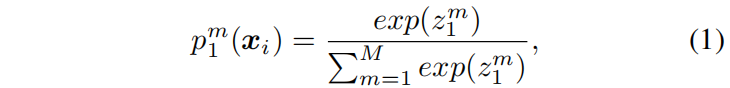where the logit $z^m$ is the output of the “softmax” layer in $Θ1$.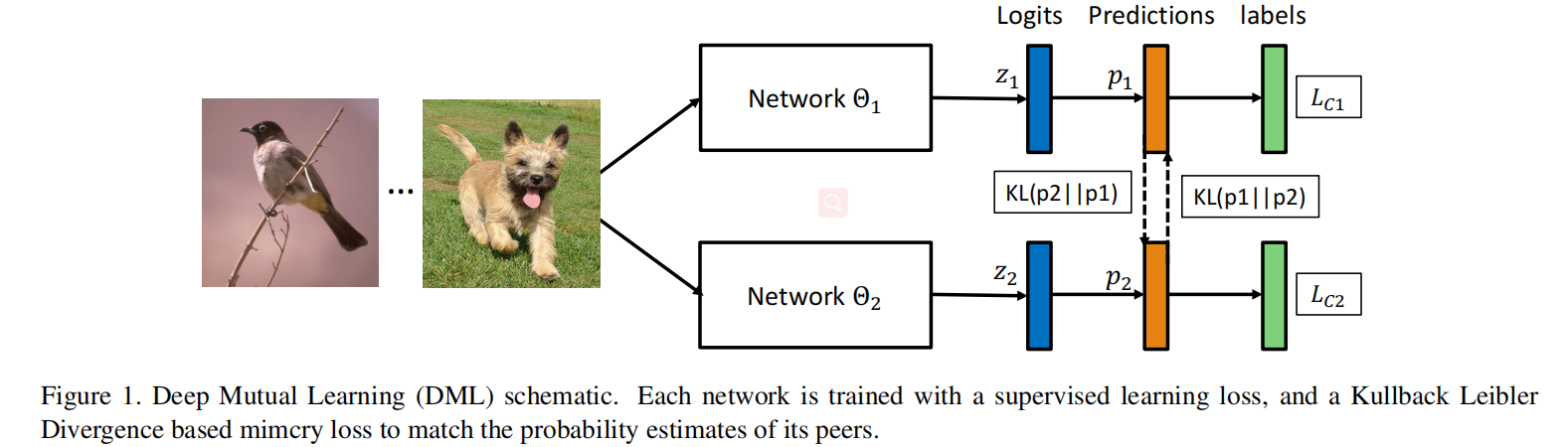For multi-class classification, the objective function to train the network $Θ1$ is defined as the cross entropy error between the predicted values and the correct labels,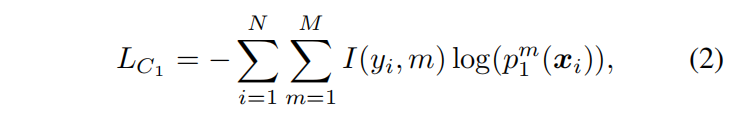with an indicator function $I$ defined as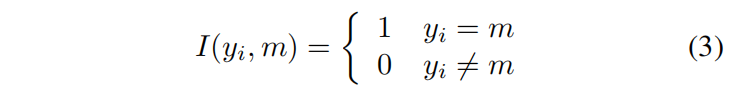To improve the generalisation performance of Θ1 on the testing instances, we use another peer network $Θ2$ to provide training experience in the form of its posterior probability p2. To quantify the match of the two network’s predictions $p_1$ and $p_2$, we use the Kullback Leibler (KL) Divergence.

The KL distance from $p1$ to $p2$ is computed as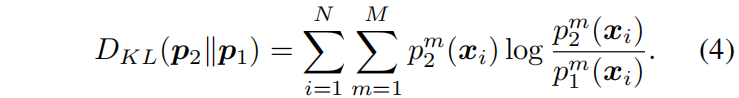The overall loss functions $L_{Θ1}$ and $L_{Θ2}$ for networks $Θ1$ and $Θ2$ respectively are thus: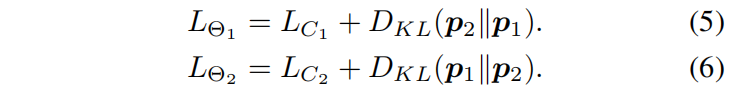Our KL divergence based mimicry loss is asymmetric, thus different for the two networks. One can instead use a symmetric Jensen-Shannon Divergence loss: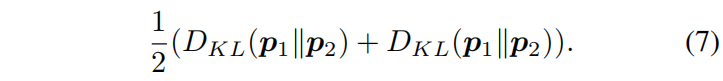However, we found empirically that whether a symmetric or asymmetric KL loss is used does not make any difference.

## 3.2. Optimisation

The mutual learning strategy is embedded in each mini-batch based model update step for both models and throughout the whole training process. At each iteration, we compute the predictions of the two models and update both networks’ parameters according to the predictions of the other. The optimisation details are summarised in Algorithm 1.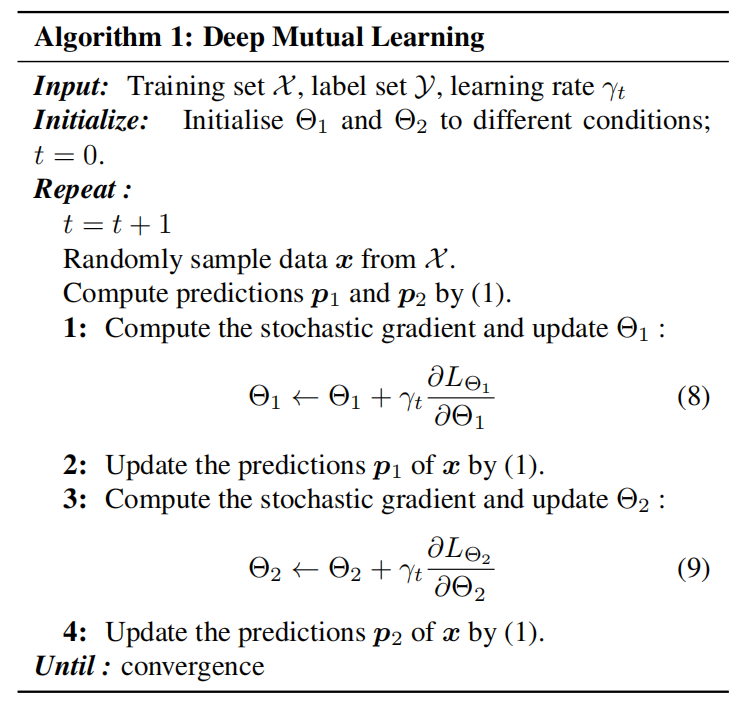## 3.3. Extension to Larger Student Cohorts

The proposed DML approach naturally extends to more networks in the student cohort. Given K networks $Θ_1, Θ_2, ..., Θ_K(K ≥ 2)$, the objective function for optimising $Θ_k,(1 ≤ k ≤ K)$ becomes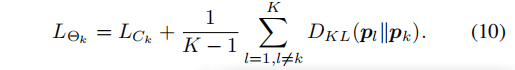With more than two networks, an interesting alternative learning strategy for DML is to take the ensemble of all the other $K − 1$ networks as a single teacher to provide a combined mimicry target, which would be very similar to the distillation approach but performed at each mini-batch model update.

Then the objective function of $Θ_k$ can be written as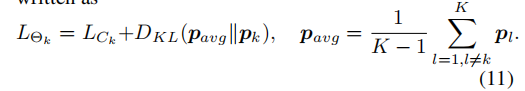## 3.4. Extension to Semi-supervised Learning

The proposed DML extends straightforwardly to semi-supervised learning. Under the semi-supervised learning setting, we only activate the cross-entropy loss for labelled data, while computing the $KL$ distance based mimicry loss for all the training data. This is because the $KL$ distance computation does not require class labels, so unlabelled data can also be used. Denote the labelled and unlabelled data as L and U, where we have X = $L∪U$, the objective function for learning network $Θ_1$ can be reformulated as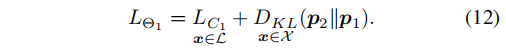# 5. Conclusion

We have proposed a simple and generally applicable approach to improving the performance of deep neural networks by training them in a cohort with peers and mutual distillation. With this approach we can obtain compact networks that perform better than those distilled from a strong but static teacher. One application of DML is to obtain compact, fast and effective networks. We also showed that this approach is also promising to improve the performance of large powerful networks, and that the network cohort trained in this manner can be combined as an ensemble to further improve performance.